Скачать презентацию What are the real challenges in data mining

0dd8ddbf68acfee414ee7a4bc2ae15fc.ppt

• Количество слайдов: 21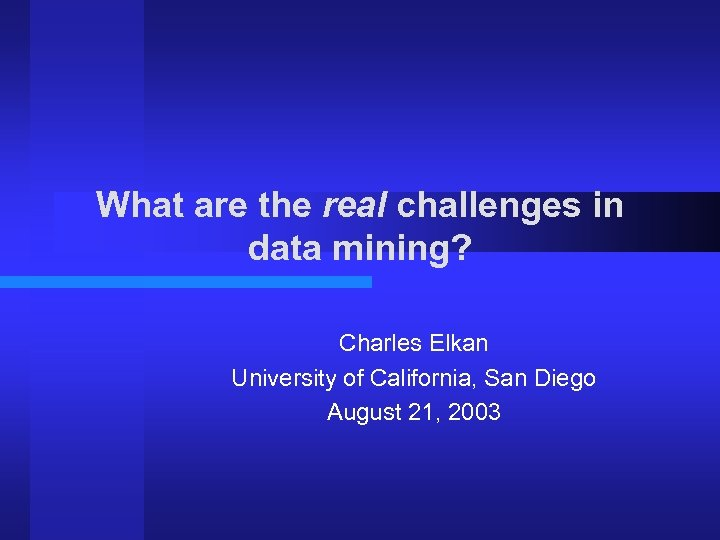What are the real challenges in data mining? Charles Elkan University of California, San Diego August 21, 2003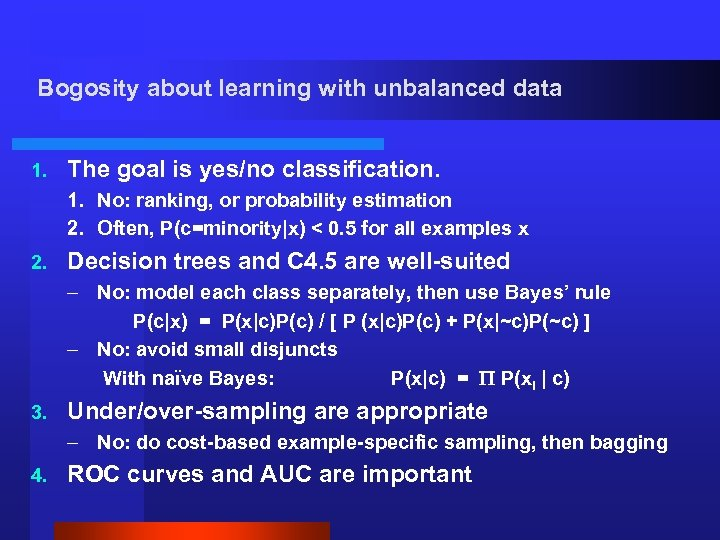Bogosity about learning with unbalanced data 1. The goal is yes/no classification. 1. No: ranking, or probability estimation 2. Often, P(c=minority|x) < 0. 5 for all examples x 2. Decision trees and C 4. 5 are well-suited – No: model each class separately, then use Bayes’ rule P(c|x) = P(x|c)P(c) / [ P (x|c)P(c) + P(x|~c)P(~c) ] – No: avoid small disjuncts With naïve Bayes: P(x|c) = P(xi | c) 3. Under/over-sampling are appropriate – No: do cost-based example-specific sampling, then bagging 4. ROC curves and AUC are important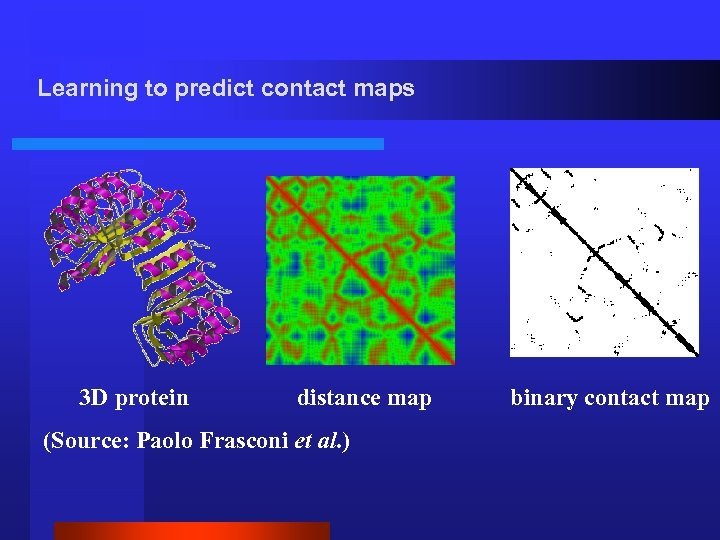Learning to predict contact maps 3 D protein distance map (Source: Paolo Frasconi et al. ) binary contact map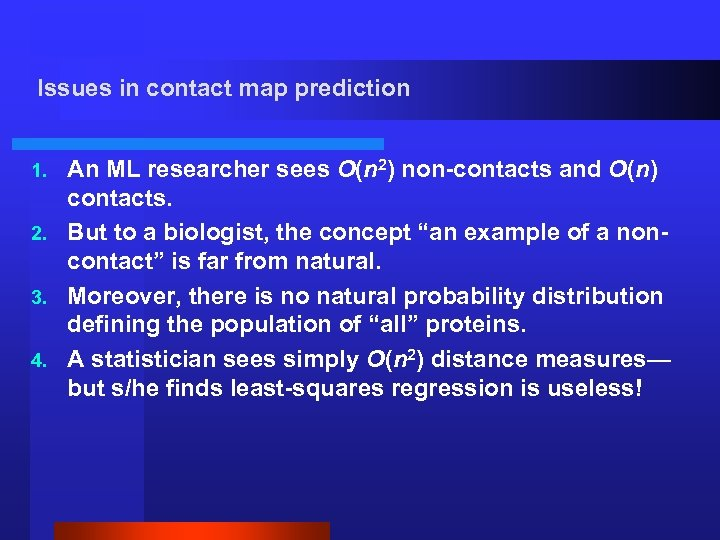Issues in contact map prediction An ML researcher sees O(n 2) non-contacts and O(n) contacts. 2. But to a biologist, the concept “an example of a non- contact” is far from natural. 3. Moreover, there is no natural probability distribution defining the population of “all” proteins. 4. A statistician sees simply O(n 2) distance measures— but s/he finds least-squares regression is useless! 1.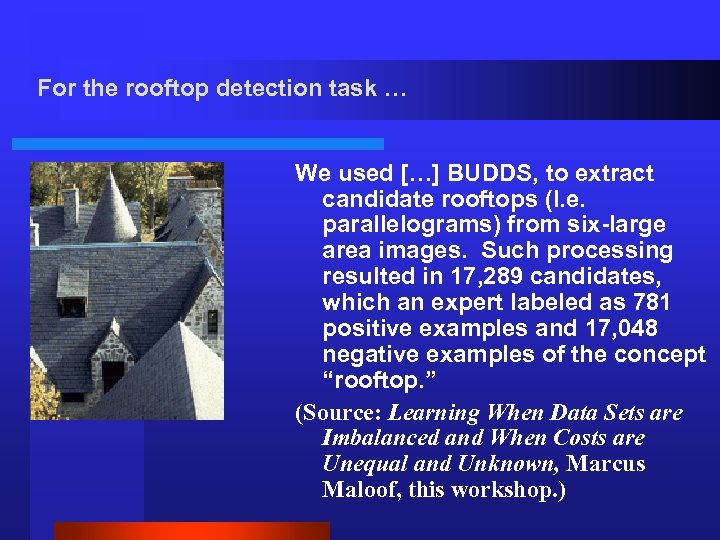For the rooftop detection task … We used […] BUDDS, to extract candidate rooftops (I. e. parallelograms) from six-large area images. Such processing resulted in 17, 289 candidates, which an expert labeled as 781 positive examples and 17, 048 negative examples of the concept “rooftop. ” (Source: Learning When Data Sets are Imbalanced and When Costs are Unequal and Unknown, Marcus Maloof, this workshop. )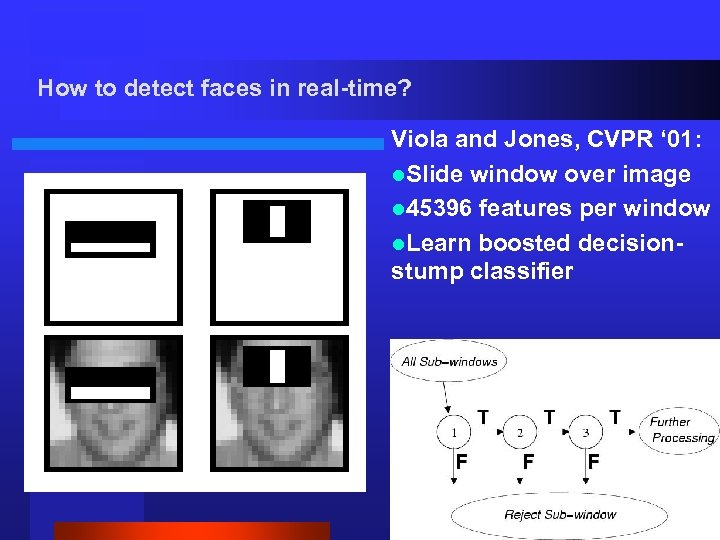How to detect faces in real-time? Viola and Jones, CVPR ‘ 01: l. Slide window over image l 45396 features per window l. Learn boosted decisionstump classifier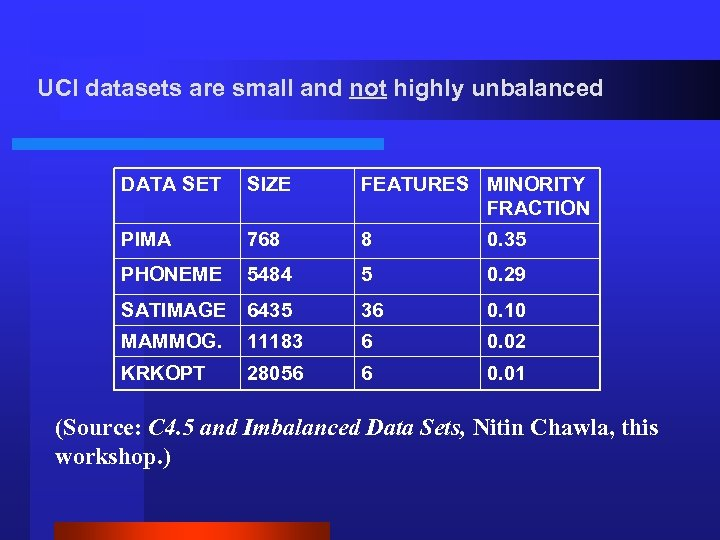UCI datasets are small and not highly unbalanced DATA SET SIZE FEATURES MINORITY FRACTION PIMA 768 8 0. 35 PHONEME 5484 5 0. 29 SATIMAGE 6435 36 0. 10 MAMMOG. 11183 6 0. 02 KRKOPT 28056 6 0. 01 (Source: C 4. 5 and Imbalanced Data Sets, Nitin Chawla, this workshop. )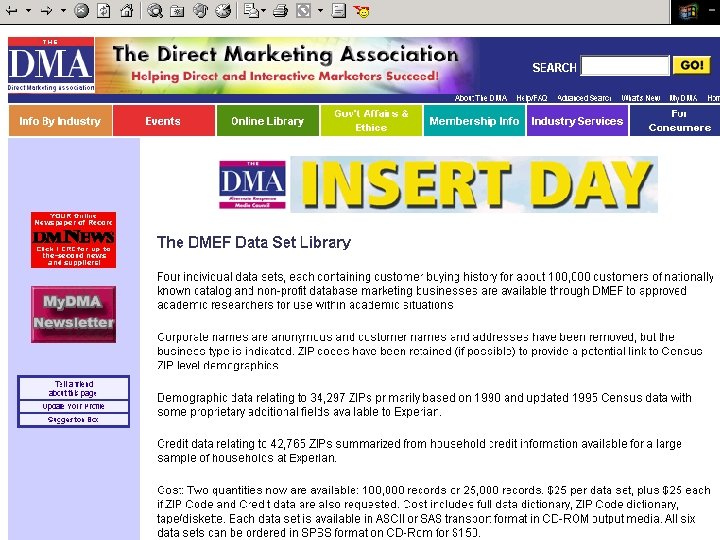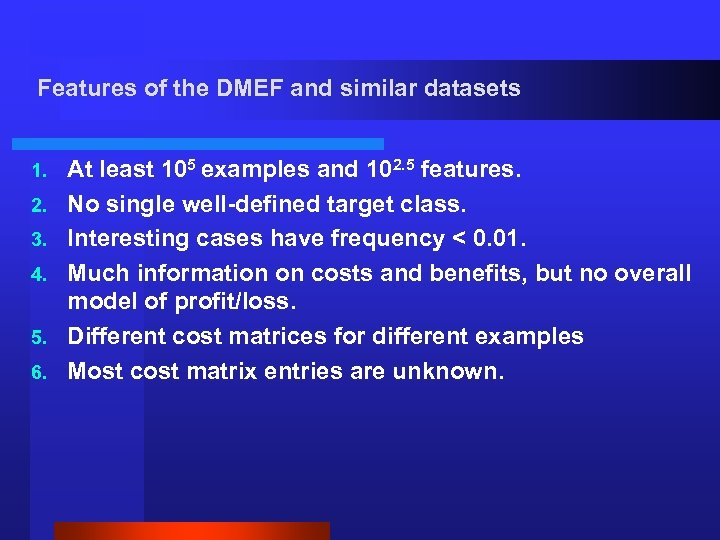Features of the DMEF and similar datasets 1. 2. 3. 4. 5. 6. At least 105 examples and 102. 5 features. No single well-defined target class. Interesting cases have frequency < 0. 01. Much information on costs and benefits, but no overall model of profit/loss. Different cost matrices for different examples Most cost matrix entries are unknown.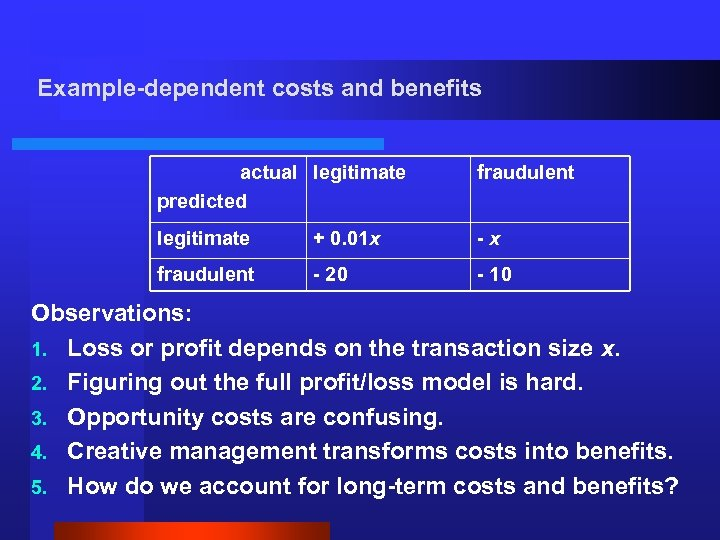Example-dependent costs and benefits actual legitimate predicted fraudulent legitimate + 0. 01 x - x fraudulent - 20 - 10 Observations: 1. Loss or profit depends on the transaction size x. 2. Figuring out the full profit/loss model is hard. 3. Opportunity costs are confusing. 4. Creative management transforms costs into benefits. 5. How do we account for long-term costs and benefits?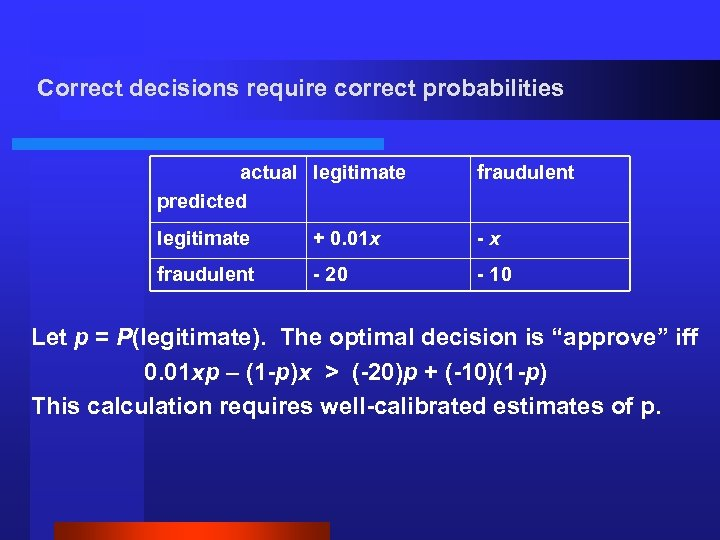Correct decisions require correct probabilities actual legitimate predicted fraudulent legitimate + 0. 01 x - x fraudulent - 20 - 10 Let p = P(legitimate). The optimal decision is “approve” iff 0. 01 xp – (1 -p)x > (-20)p + (-10)(1 -p) This calculation requires well-calibrated estimates of p.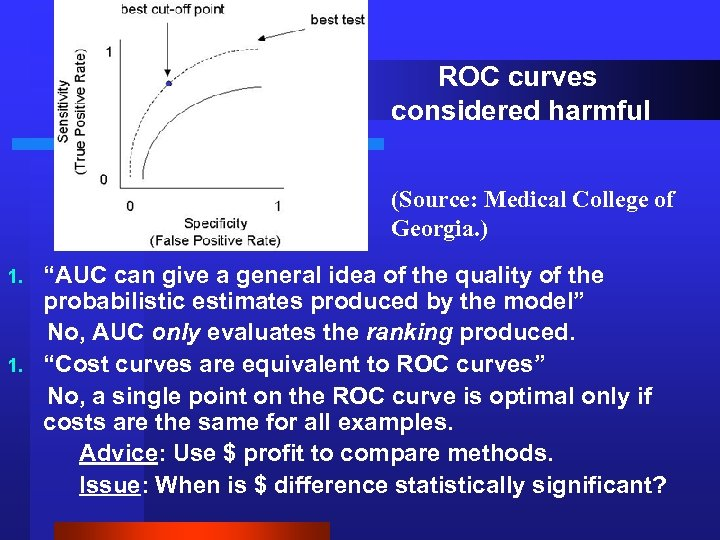ROC curves considered harmful (Source: Medical College of Georgia. ) “AUC can give a general idea of the quality of the probabilistic estimates produced by the model” No, AUC only evaluates the ranking produced. 1. “Cost curves are equivalent to ROC curves” No, a single point on the ROC curve is optimal only if costs are the same for all examples. Advice: Use \$ profit to compare methods. Issue: When is \$ difference statistically significant? 1.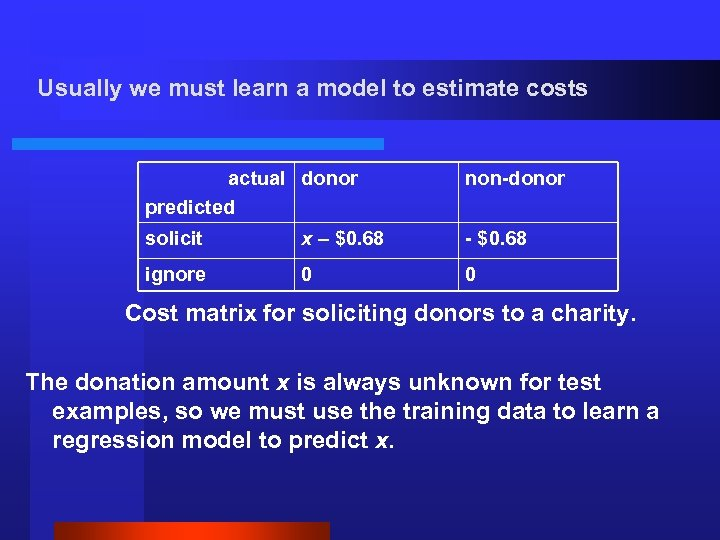Usually we must learn a model to estimate costs actual donor predicted non-donor solicit x – \$0. 68 - \$0. 68 ignore 0 0 Cost matrix for soliciting donors to a charity. The donation amount x is always unknown for test examples, so we must use the training data to learn a regression model to predict x.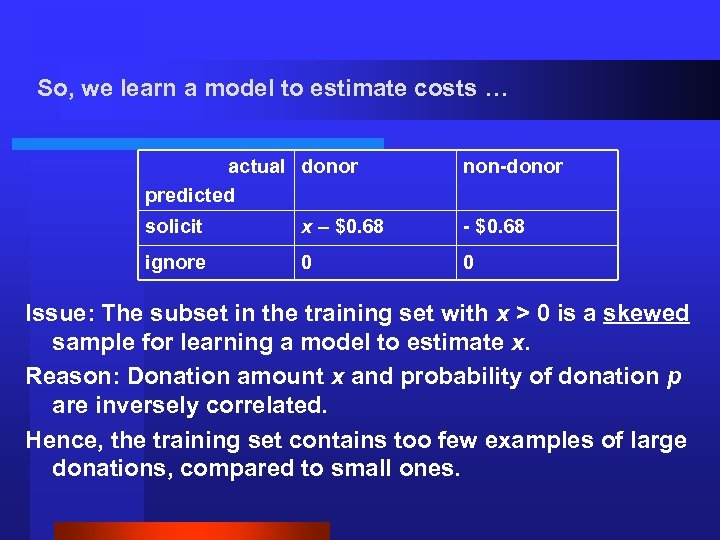So, we learn a model to estimate costs … actual donor predicted non-donor solicit x – \$0. 68 - \$0. 68 ignore 0 0 Issue: The subset in the training set with x > 0 is a skewed sample for learning a model to estimate x. Reason: Donation amount x and probability of donation p are inversely correlated. Hence, the training set contains too few examples of large donations, compared to small ones.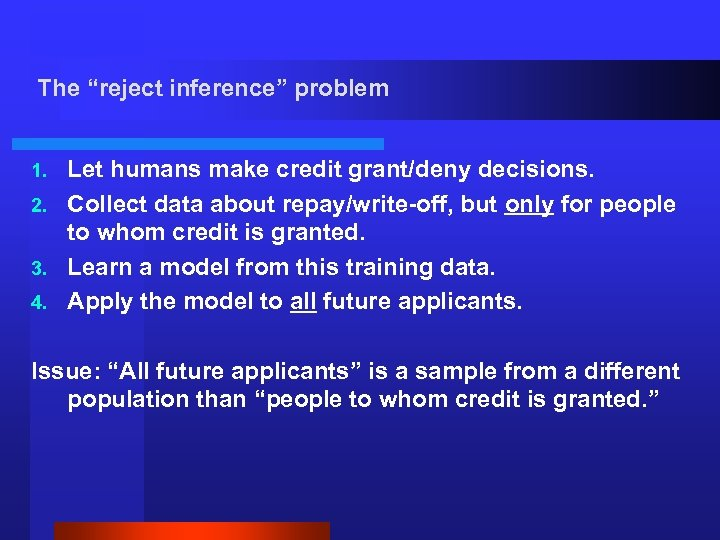The “reject inference” problem Let humans make credit grant/deny decisions. 2. Collect data about repay/write-off, but only for people to whom credit is granted. 3. Learn a model from this training data. 4. Apply the model to all future applicants. 1. Issue: “All future applicants” is a sample from a different population than “people to whom credit is granted. ”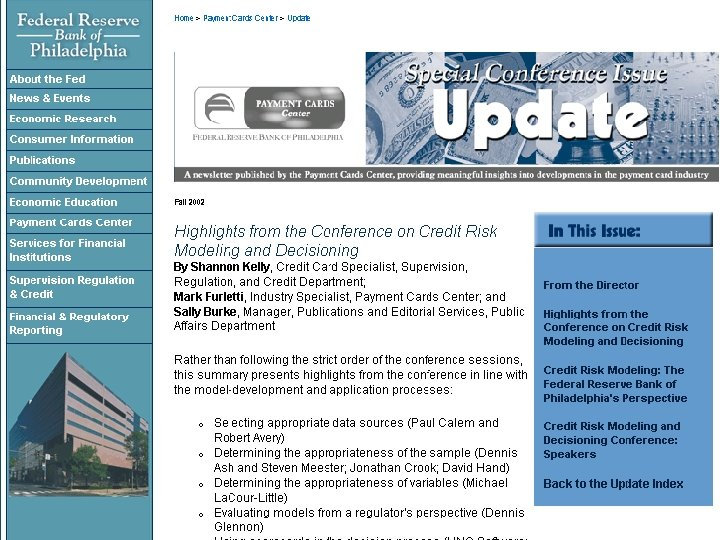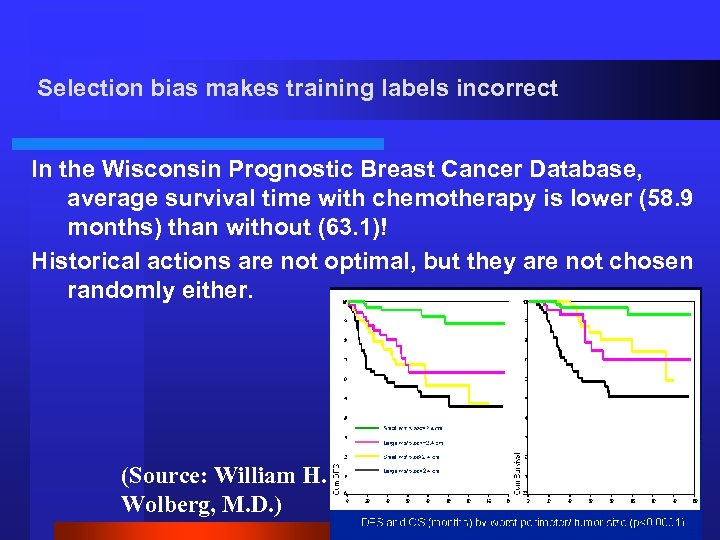Selection bias makes training labels incorrect In the Wisconsin Prognostic Breast Cancer Database, average survival time with chemotherapy is lower (58. 9 months) than without (63. 1)! Historical actions are not optimal, but they are not chosen randomly either. (Source: William H. Wolberg, M. D. )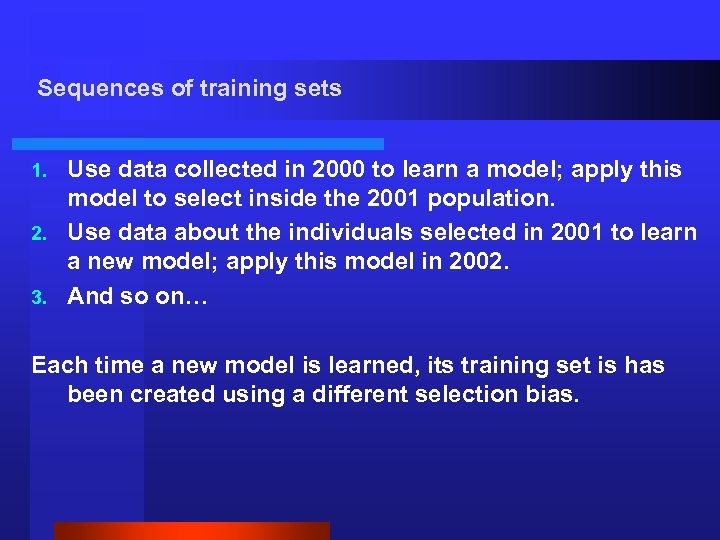Sequences of training sets Use data collected in 2000 to learn a model; apply this model to select inside the 2001 population. 2. Use data about the individuals selected in 2001 to learn a new model; apply this model in 2002. 3. And so on… 1. Each time a new model is learned, its training set is has been created using a different selection bias.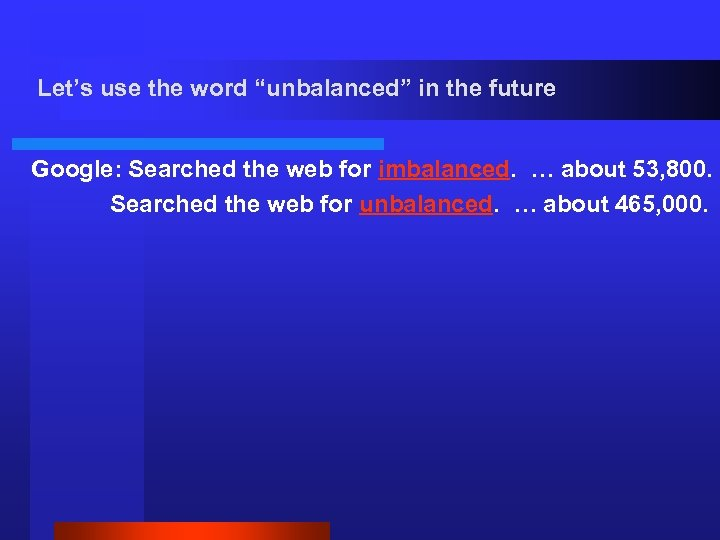Let’s use the word “unbalanced” in the future Google: Searched the web for imbalanced. … about 53, 800. Searched the web for unbalanced. … about 465, 000.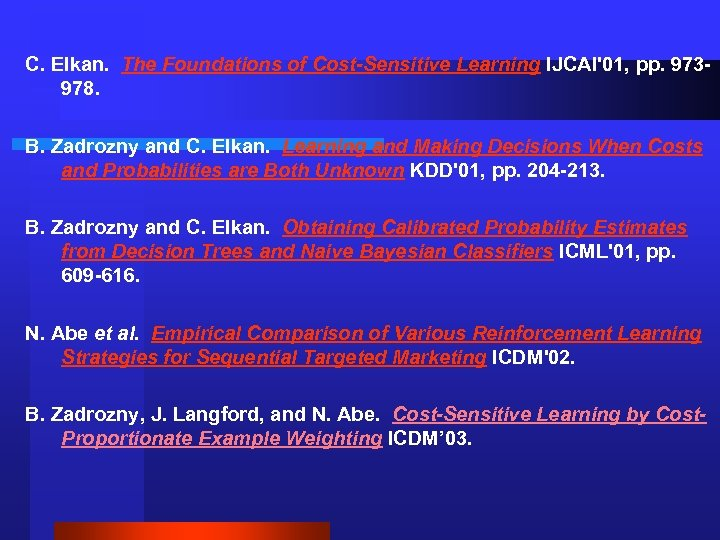C. Elkan. The Foundations of Cost-Sensitive Learning IJCAI'01, pp. 973978. B. Zadrozny and C. Elkan. Learning and Making Decisions When Costs and Probabilities are Both Unknown KDD'01, pp. 204 -213. B. Zadrozny and C. Elkan. Obtaining Calibrated Probability Estimates from Decision Trees and Naive Bayesian Classifiers ICML'01, pp. 609 -616. N. Abe et al. Empirical Comparison of Various Reinforcement Learning Strategies for Sequential Targeted Marketing ICDM'02. B. Zadrozny, J. Langford, and N. Abe. Cost-Sensitive Learning by Cost. Proportionate Example Weighting ICDM’ 03.